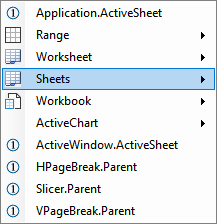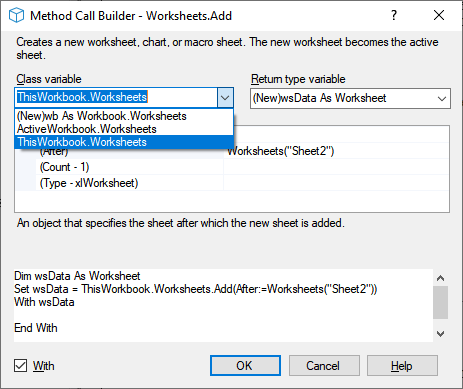﻿ Set Worksheet using VBA# Declare and Set Worksheet

To be able to operate on a worksheet using VBA you have to specify which worksheet. Below discusses the many ways you can do this. The sample code declares and sets variables for the worksheet as a best practice. If you use the Code VBAadd-in using Set will add the declaration automatically. The below sections give an overview of the ways to Set a worksheet.

Note
It is possible to not use a variable in your code, but to operate directly on available methods that return a worksheet. As an example `Application.ActiveSheet.Name` will get you the name of the active worksheet - no need for a variable. However it is in most cases better to declare a variable in your code to refer to the worksheet because:
• You are likely to have to refer to the worksheet you work on more than once in your code, in which case a variable often is shorter.
• The name of the variable can make it more clear what its role is. It can be as simple as `ws` if there is only one workbook involved, or maybe wbActiveSheet if your code works on any active sheet.
• It allows you to be very explicit about which sheet you really mean - any worksheet that happens to be on top, one with a specific name or position in workbook, in which workbook, etcetera.

## Set a Worksheet you just added

You can add a new worksheet and directly start working on it. Code VBA has builders which make it easy to tweak the resulting code.## Set Worksheet in a specified workbook - by name

If you write macros for a specific line of business, your worksheet actions will generally assume a specific (type of) workbook as it's context. As an example, below code could be included in an Excel file with a worksheet called Locations. If your macro would involve more worksheets, you would probably name the variable wsLocations.

``````Dim wb As Workbook: Set wb = ThisWorkbook
Dim ws As Worksheet
Set ws = wb.Sheets("Locations")
If ws Is Nothing Then
Exit Sub 'possible way of handing no worksheet was set
End If``````

Returns Nothing if no sheet exists with that name. In fact any approach that tries to return a worksheet may return Nothing, so you should always check if a valid worksheet was set.

Note
ws and wb are commonly used short names and prefixes for Worksheet and Workbook

## Set Worksheet in general purpose macros

If you want your macro to be usable in any workbook, you probably want it to work on any active sheet (= the one on top) - for example, clear its contents.

``````Dim wsActiveSheet As Worksheet
Set wsActiveSheet = Application.ActiveSheet
wsActiveSheet.UsedRange.Clear``````

### ActiveSheet

ActiveSheet returns the active sheet (the sheet on top) in the active workbook or active window. Above code shows the use of the default use: `Application.ActiveSheet`

#### ActiveSheet in Workbook

If your macro only is to be used in a specific workbook, for example one that it has just opened, use the more specific

``````Dim wb As Workbook: Set wb =
Dim wsActiveSheet As Worksheet
Set wsActiveSheet = wb.ActiveSheet``````

#### ActiveSheet in Window

If the workbook appears in more than one window, `wb.ActiveSheet` may be different in different windows. In that case use:

``````Dim wnd As Window: Set wnd =
Dim wsActiveSheet As Worksheet
Set wsActiveSheet = wnd.ActiveSheet``````

## Obtaining the worksheet from a property of another object

### Range.Worksheet

``````Dim rng As Range: Set rng =
Dim wsWorksheet As Worksheet
Set wsWorksheet = rng.Worksheet``````

### Next and Previous Worksheet

This way of setting a worksheet is available for both Worksheet and Chart objects

``````Dim ws As Worksheet: Set ws =
Dim wsNext As Worksheet
Set wsNext = ws.Next``````
``````Dim ws As Worksheet: Set ws =
Dim wsPrevious As Worksheet
Set wsPrevious = ws.Previous``````

### Parent property of a PageBreak object

``````Dim hpgbr As HPageBreak: Set hpgbr =
Dim wsParent As Worksheet
Set wsParent = hpgbr.Parent``````